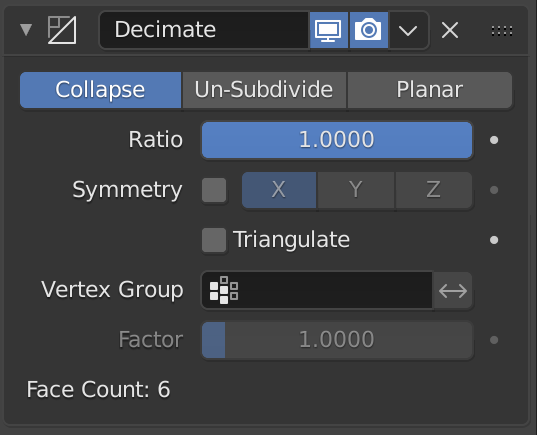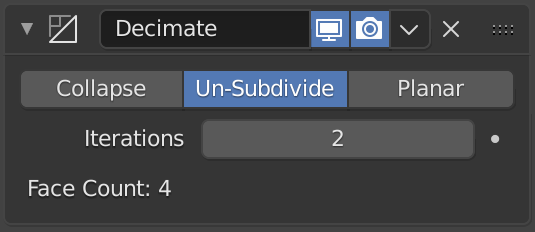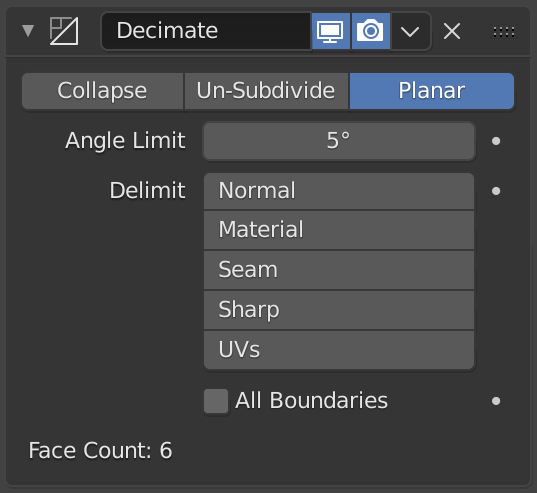# Decimate Modifier¶

The Decimate modifier allows you to reduce the vertex/face count of a mesh with minimal shape changes.

This is not usually used on meshes which have been created by modeling carefully and economically (where all vertices and faces are necessary to correctly define the shape). But if the mesh is the result of complex modeling, sculpting and/or applied Subdivision Surface/ Multiresolution modifiers, the Decimate one can be used to reduce the polygon count for a performance increase, or simply remove unnecessary vertices and edges.

Unlike the majority of existing modifiers, this one does not allow you to visualize your changes in Edit Mode.

## Options¶

### Decimate Type¶

#### Collapse¶The Decimate modifier in Collapse mode.

Merges vertices together progressively, taking the shape of the mesh into account.

Ratio

The ratio of faces to keep after decimation.

• On 1.0: the mesh is unchanged.
• On 0.5: edges have been collapsed such that half the number of faces remain (see note below).
• On 0.0: all faces have been removed.

Bemerkung

Although the Ratio is directly proportional to the number of remaining faces, triangles are used when calculating the ratio.

This means that if your mesh contains quads or other polygons, the number of remaining faces will be larger than expected, because those will remain unchanged if their edges are not collapsed.

This is only true if the Triangulate option is disabled.

Vertex Group

A vertex group that controls what parts of the mesh are decimated.

Factor
The amount of influence the Vertex Group has on the decimation.
Triangulate
Keeps any resulting triangulated geometry from the decimation process.
Symmetry
Maintains symmetry on a single axis.

#### Un-Subdivide¶The Decimate modifier in Un-Subdivide mode.

It can be thought of as the reverse of subdivide. It attempts to remove edges that were the result of a subdivide operation. It is intended for meshes with a mainly grid-based topology (without giving uneven geometry). If additional editing has been done after the subdivide operation, the results may be unexpected.

Iterations
The number of times to perform the un-subdivide operation. Two iterations is the same as one subdivide operation, so you will usually want to use even numbers.

#### Planar¶The Decimate modifier in Planar mode.

It reduces details on forms comprised of mainly flat surfaces.

Angle Limit
Dissolve geometry which form angles (between surfaces) higher than this setting.
All Boundaries
When enabled, all vertices along the boundaries of faces are dissolved. This can give nicer results when using a high Angle Limit.
Delimit

Prevent dissolving geometry in certain places.

Normal
Does not dissolve edges on the borders of areas where the face normals are reversed.
Material
Does not dissolve edges on the borders of where different materials are assigned.
Seam
Does not dissolve edges marked as seams.

### Further Options¶

Face Count
This label shows the number of remaining faces as a result of applying the Decimate modifier.